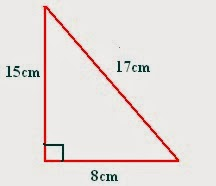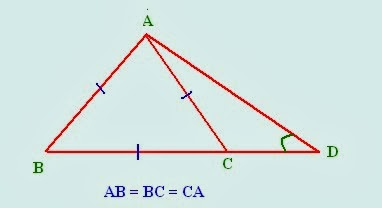In my previous session, i discussed some important questions of General Intelligence and Reasoning for upcoming SSC CGL Tier I exam. Today, I'll discuss questions of Quantitative Aptitude section which will give you an outlook of exam pattern. As we all know, this section also includes data interpretation, I will discuss its questions in parts.

## Quantitative Aptitude

1. If  S_1 = 2, S_2 = 3 and S_(n+3) = S_(n+1) + S_(n+2), then what will be the fifth term of the sequence?

Solution:  Sequence and Series
Given that S_1 = 2, S_2 = 3

To find: S_5

Now, given pattern of sequence is  S_(n+3) = S_(n+1) + S_(n+2)

If n = 2,
S_(2+3) = S_(2+1) + S_(2+2)
S_5 = S_3 + S_4

Similarly,
If n = 0,
S_3 = S_1 + S_2 and
If n = 1,
S_4 = S_2 + S_3

Putting values of S_3 and S_4 in equation of S_5

S_5 = S_3 + S_4
⇒S_5 = S_3 + S_2 + S_3
⇒S_5 =  2(S_1 + S_2) + S_2
⇒S_5 = 2(S_1) + 3(S_2)
⇒S_5 = 2(2) + 3(3)
⇒S_5 = 4+ 9 = 13

2. A group of 12 men and 16 women can do a piece of work in 5 days and a group of 13 men and 24 women can do the same work in 4 days. How many days will 7 men and 10 women take to finish the same work?

Solution: Time and Work
12 Men + 16 Women i.e. 12 M + 16 W takes 5 days
and 13 M + 24 W takes 4 days

I will solve this question using per day work,

In one day, work is done by : 5(12 M + 16 W) and
4(13 M + 24 W)

Therefore, 5(12 M+ 16 W) = 4(13 M + 24W)
⇒ 1M = 2W

So, equation becomes: 12M + 16 W = 40 W (takes 5 days)

To find: 7 M + 10 W = 24 W

Using basic formula, M_1 times D_1 = M_2 times D_2

D_2 = (40 times 5)/ (24) = 8.33 days

3. Base of a right angled triangle is 8 cm and hypotenuse is 17 cm. Find the area of triangle.

Solution: Using Pythagoras theorem:

(Hypotenuse)^2 = (base)^2 + (perpendicular)^2

(17)^2 = 8^2 + P^2
⇒ 289 = 64 + P^2
⇒P = 15 cmNow area of right angled triangle = 1/2 times Base times Perpendicular

Area = (1/2) times 8 times 15
⇒ 60 cm^2

4. Rs 1100 is divided among A, B and C in such a way that the ratio of amounts of A and B is 3: 2 and that of B and C is 5: 4. Find the amounts each received?

Ratios are:
A: B = 3: 2
B: C = 5: 4

A: B: C = 15 : 10 : 8

A's share = (1100 times 15)/ (15+10+8)
⇒(1100 times 15)/ 33
⇒Rs 500

B's share = (1100 times 10)/ 33
⇒ Rs 333.33
C's share = (1100 times 8)/ 33
⇒ Rs 266.66

5. If x is 5 more than y and sum of squares of x and y is 55, then what will be the product of x and y?

Solution: Given that: x = y + 5........................(1)

x^2 + y^2 = 55 .................(2)
To find: xy

We know that, (x-y)^2 = x^2 + y^2 - 2xy
From eqn 1 and 2

5^2 = 55 - 2 xy

⇒ 2xy = 30
⇒ xy = 15

6. In the following figure, Find angle D, where, AB = BC = CA = CDSolution: Geometry
As sides AB = BC = CA
Therefore, angle ACB = ABC = BAC = 60^o (sum of all angles = 180^o)

angle ACD = 180^o - 60^o
⇒ angle ACD = 120^o

Now, as sides AC and CD ARE equal,

Also, CAD + ADC + ACD = 180^o
So, angle D = 30^o

7. If x + 1/x = 2, then what will be the value of x^2 + 1/(x^2)?

Solution: Using formula, (x+y)^2 = x^2 + y^2 + 2xy

Here, y = 1/x
Therefore, (x + (1/x))^2 = x^2 + 1/(x^2) +2 (x)(1/x)

⇒  x^2 + 1/(x^2) = 2^2 - 2 = 2

8. What will be the volume of right circular cylinder if radius = 2 cm and height = 2.5 cm?

Solution: Volume of right circular cylinder = pi r^2 h

r = 2 cm
h = 2.5 cm

Therefore, Volume = (3.14) times 4 times (2.5)
⇒ 31.4 cm

Soon i will update more questions for practice.
For General awareness and Reasoning, Check following article:

Important Questions of Quantitative Aptitude for SSC CGL Tier I-2014Reviewed by Jasleen Behl on Saturday, February 08, 2014 Rating: 5